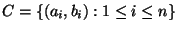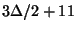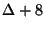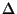Next: MINIMUM SEPARATING SUBDIVISION Up: Miscellaneous Previous: MINIMUM BEND NUMBER   Index

### MINIMUM LENGTH TRIANGULATION

• INSTANCE: Collectionof pairs of integers giving the coordinates of n points in the plane.

• SOLUTION: A triangulation of the set of points represented by C, i.e., a collection E of non-intersecting line segments each joining two points in C that divides the interior of the convex hull into triangular regions.

• MEASURE: The discrete-Euclidean length of the triangulation, i.e.,• Good News: In APX .

• Comment: Note that the problem is not known to be NP-complete. The Steiner variation in which the point set of E must be a superset of C is approximable within 316 .

Triangulating planar graphs while minimizing the maximum degree is approximable so that the maximum degree is bounded byfor general graphs andfor triconnected graphs, whereis the degree of the graph .

• Garey and Johnson: OPEN12

Viggo Kann
2000-03-20